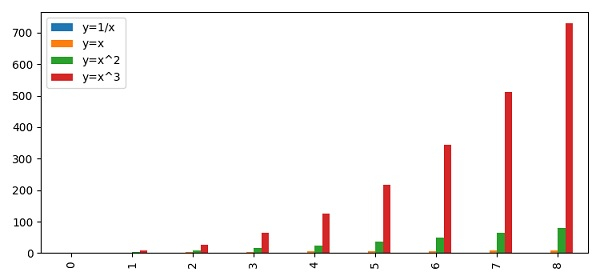# Plot multiple columns of Pandas dataframe on the bar chart in Matplotlib

To plot multiple columns of a Pandas dataframe on the bar chart in matplotlib, we can take the following steps −

• Make a dictionary of different keys, between 1 to 10 range.

• Make a dataframe using Pandas dataframe.

• Create a bar plot, using the plot() method with kind=”bar”.

• To display the figure, use the show() method.

## Example

import pandas as pd
from matplotlib import pyplot as plt
plt.rcParams["figure.figsize"] = [7.50, 3.50]
plt.rcParams["figure.autolayout"] = True
d = {
'y=1/x': [1 / i for i in range(1, 10)],
'y=x': [i for i in range(1, 10)],
'y=x^2': [i * i for i in range(1, 10)],
'y=x^3': [i * i * i for i in range(1, 10)]
}
df = pd.DataFrame(d)
df.plot(kind='bar')
plt.show()

## Output